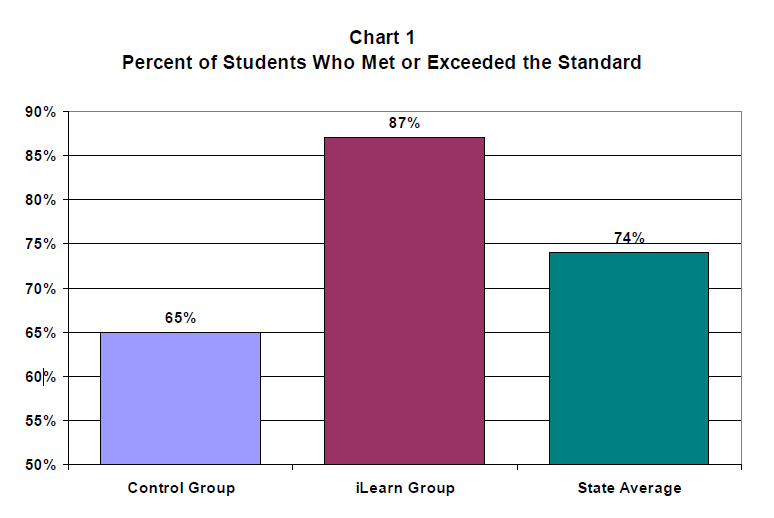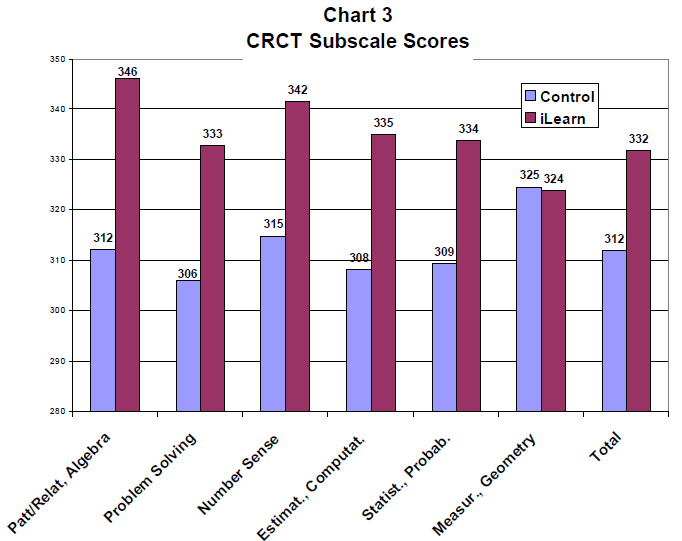# Achievement Study Results

## iLearn Math Produces Exceptionally Large Gains in Student Achievement in One School Year

In the fall of 2004, iLearn Math was implemented in the sixth grade of a middle school in a rural area of Georgia. A total of 101 students participated, 51 of whom used iLearn Math. iLearn was used as primary instruction for these students for the entire school year. That is, their only math instruction was the classroom in which iLearn was used as the sole method of instruction. The remaining 50 students in the sixth grade received their normal classroom instruction (without iLearn Math). These students served as a “control” group to evaluate the impact of using iLearn Math with the other group.

At the start of the school year, all students were given a pretest. The scores on the pretest for the two groups were statistically the same. At the end of the school year, the impact of using iLearn was assessed by comparing the scores for the two groups on the Georgia Criterion Referenced Competency Test (CRCT) administered annually to all students in Georgia through eighth grade. Note that this is the toughest standard for evaluating the impact of an intervention, since the CRCT is an independent, third-party test developed and implemented by a State Department of Education. It is used for assessing the schools. Annual Yearly Progress (AYP) under the No Child Left Behind Law (NCLB). The results for the CRCT are shown below.As seen in Chart 1, using iLearn Math made an exceptionally large impact on math scores. The percentage of iLearn Math students who met or exceeded the standard on the CRCT was 87%, whereas the percentage of control group students was 65%. Thus, the use of iLearn Math resulted in an increase of 22 percentage points on the CRCT in a single year. The average value for the state using the same measure was 74%. The students who did not receive iLearn Math scored 9 percentage points below the state average. The students who did receive iLearn Math scored 13 percentage points above the state average. This is an exceptionally large gain for a single school year.

Another way of viewing the results is in terms of the failure rate on the CRCT. The failure rate was reduced from 35% of the Control Group students to 13% of the iLearn Group, a reduction of 63%. Under the NCLB guidelines, the percent of students failing to meet the standard should be 0% by the year 2014. Thus, 63% of the gain required by year 2014 (10 years from now) was achieved in a single year!

Chart 2 below indicates that iLearn Math also had a similar large impact on scores of students near the upper end of the range. The percentage of iLearn Math students exceeding the standard increased by 280%, from 10% for the control group to 28% for the iLearn group.These results indicate that iLearn Math can be an extremely powerful tool for improving math achievement, and can greatly accelerate the gains necessary to meet the guidelines of the NCLB Act.

One question that is often raised about instruction delivered by a computer is whether it produces .real understanding., not simply rote learning. The data below sheds light on this issue. Chart 3 shows the data for both groups by subscale on the CRCT. They are arranged in order of increasing difference between the iLearn Math Group and the Control Group from left to right.The leftmost comparison for Patterns, Relationships, and Algebra showed the greatest difference between the iLearn Group and the Control Group. The second largest difference between the Groups was for Problem Solving and the third largest difference was for Number Sense. The subscale for Computation showed the fourth largest difference between the Groups. The order of these differences is significant because it indicates that the greatest impact of using iLearn Math was NOT on the Computation subscale, as one would expect if the students were simply learning computation rules in rote fashion, devoid of any real understanding. Instead, the greatest gains were with subscales that typically indicate conceptual understanding of the content. This is clear evidence that iLearn Math greatly improves students. conceptual understanding of the content, not just rote proficiency in computation.